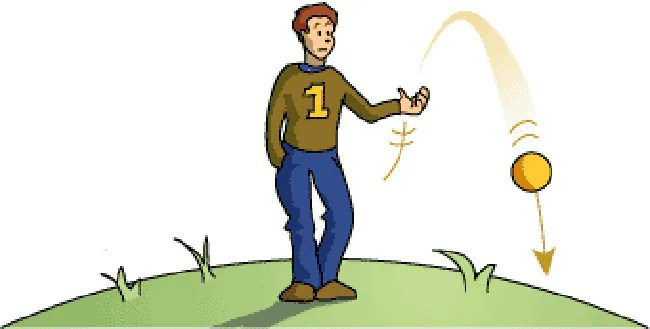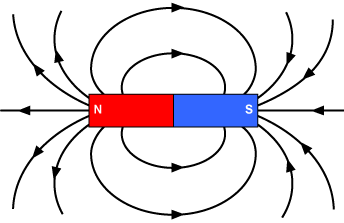Yourclasses: Best Preparation website for SSC, Banking & Railway Exams

# Science : Force (Part-1)

### What is force?

Force is a push or a pull on an object.

It changes or tends to change the state or shape of an object.

• I. Unit is Newton (N)
• It is a vector quantity
• Whenever we are pushing, pulling, lifting bending, twisting, tearing we are exerting a force.

### Force can –

• Make a stationary body move.
• Change the speed of a body.
• Change the direction of motion of a body
• Change the size or shape of the body

### Here are some important forces which you are familiar with –

• Gravitational force – Force due to gravitational pull.• Frictional force – Force which opposes motion.• Electrical force – Force of attraction or repulsion between electrical charges.• Magnetic force – Force of attraction or repulsion between the poles of magnets.• Contact force – Force of repulsion when two objects are pressed together.## Questions asked in previous years.

Q.1       Which of the following force is a virtual force?

(a) Frictional force

(b) Centripetal force

(c) Centrifugal force

(d) Nuclear force

Ans. (a)  Centrifugal force is an inertial force that is acted upon a body moving on a circular path.

• It acts outwards from the centre of rotation.

Q.2       Water drops cannot stick to the oily surface due to –

(a) Atmospheric pressure

(b) Viscosity of water

(d) Surface tension

Ans. (c) Water drops cannot stick to the oily surface due to lack of adhesive force.

Adhesive force – Adhesive forces are the attractive forces between different molecules.

Q.3       Ball pen functions on the principal of –

(a) Viscosity

(b) Boyle’s law

(c) Gravitational force

(d) Surface tension

Ans.(d) Ball pen works surface tension

Surface tension:- The force that causes the molecules on the surface of a liquid to be bound together and form a layers.

Q.4       Upward force on a floating body is called –

(a) Buoyancy                (b) Jerk                         (c) Gravitational            (d) Floatation

Ans. (a) Buoyancy force acts upon an object that is floating on a fluid.

Q.5       Which contact force is responsible for changing the state of motion of an object?

(a) Magnetic force

(b) Frictional Force

(c) Electrical force

(d) electro-Magnetic Force

Ans. (b)   Frictional force is responsible for changing the state of motion of an object.

#### You may also like...

This site uses Akismet to reduce spam. Learn how your comment data is processed.